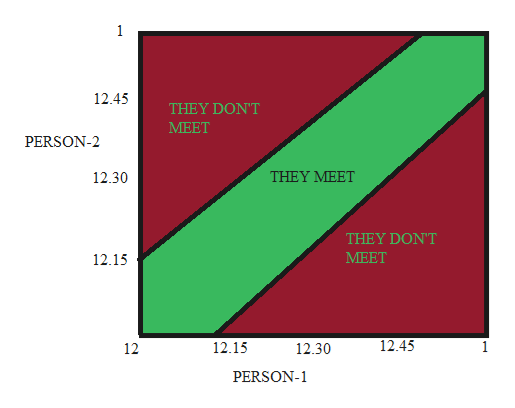# 1. Two businessmen agree to meet for lunch between 12 and 1. They are not surethey will be able to make it, so they both agree that the first person to arrive willwait for 15 minutes to see if the other person shows up. After 15 minutes, the firstperson to arrive will leave if the other person is not there. What is the probabilitythat the two businessmen will have lunch together?2.A coin is weighted so that heads (H) is twice as likely to appear as tails(T). FindP(T) and P(H)3.Suppose A and B are events such that P(A) = 0.6, P(B) = 0.3, and P(A∩B) = 0.2.Find the probability that(a) A does not occur(b) A or B occurs(c) neither A nor B occurs4.Suppose p(A) = p. The odds that A occurs is a to b. Write a formula for pPlease be as detailed as possible even with the fractions. Thank you!

Question
47 views

1. Two businessmen agree to meet for lunch between 12 and 1. They are not sure
they will be able to make it, so they both agree that the first person to arrive will
wait for 15 minutes to see if the other person shows up. After 15 minutes, the first
person to arrive will leave if the other person is not there. What is the probability
that the two businessmen will have lunch together?

2.A coin is weighted so that heads (H) is twice as likely to appear as tails(T). Find
P(T) and P(H)

3.Suppose A and B are events such that P(A) = 0.6, P(B) = 0.3, and P(A∩B) = 0.2.
Find the probability that
(a) A does not occur
(b) A or B occurs
(c) neither A nor B occurs

4.Suppose p(A) = p. The odds that A occurs is a to b. Write a formula for p

Please be as detailed as possible even with the fractions. Thank you!

check_circle

star
star
star
star
star
1 Rating
Step 1

Hi! You seem to have uploaded many questions at a time. So, only first three questions are being answered since nothing is specified. If you need the solution for the last part then kindly re-post the question with specification of that particular question.

Step 2

Two businessmen agree to meet for lunch between 12 and 1. They are not sure

they will be able to make it, so they both agree that the first person to arrive will

wait for 15 minutes to see if the other person shows up. After 15 minutes, the first

person to arrive will leave if the other person is not there.

Here we need to find out the probability that the two businessmen will have lunch together.

We will answer this question graphically.

Step 3

Let us draw person-1 in x-axis and person-2 in y-axis.

And we graphically denote the possible area where person-1 and person-2 can meet.

Note:

Calculation of the area is done by taking up their possible arriva...help_outlineImage Transcriptionclose1 12.45 THEY DON'T МЕЕТ PERSON-2 THEY MEET 12.30 THEY DON'T МЕЕТ 12.15 12.45 12.15 1 12.30 12 PERSON-1 fullscreen

### Want to see the full answer?

See Solution

#### Want to see this answer and more?

Solutions are written by subject experts who are available 24/7. Questions are typically answered within 1 hour.*

See Solution
*Response times may vary by subject and question.
Tagged in

### Basic Probability